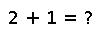# linreg(x,y)

linreg(x, y) -> a, b

Perform linear regression. Returns `a` and `b` such that `a + b*x` is the closest straight line to the given points `(x, y)`, i.e., such that the squared error between `y` and `a + b*x` is minimized.

Example:

``````using PyPlot
x = [1.0:12.0;]
y = [5.5, 6.3, 7.6, 8.8, 10.9, 11.79, 13.48, 15.02, 17.77, 20.81, 22.0, 22.99]
a, b = linreg(x, y)          # Linear regression
plot(x, y, "o")              # Plot (x, y) points
plot(x, [a+b*i for i in x])  # Plot line determined by linear regression``````

## Examples

Checking you are not a robot: